# Tetrahedron

Go back to  'Solid Shapes'

 1 Introduction to Tetrahedron 2 What is a Tetrahedron? 3 Tetrahedron Properties 4 Tetrahedron Formula 5 Solved Examples on Tetrahedron 6 Important Notes on Tetrahedron 7 Practice Questions on Tetrahedron 8 Challenging Questions on Tetrahedron 10 Maths Olympiad Sample Papers 11 Frequently Asked Questions (FAQs)

We at Cuemath believe that Math is a life skill. Our Math Experts focus on the “Why” behind the “What.” Students can explore from a huge range of interactive worksheets, visuals, simulations, practice tests, and more to understand a concept in depth.

Book a FREE trial class today! and experience Cuemath’s LIVE Online Class with your child.

## Introduction to Tetrahedron

A tetrahedron is one of the five platonic solids.

It has triangles as its faces.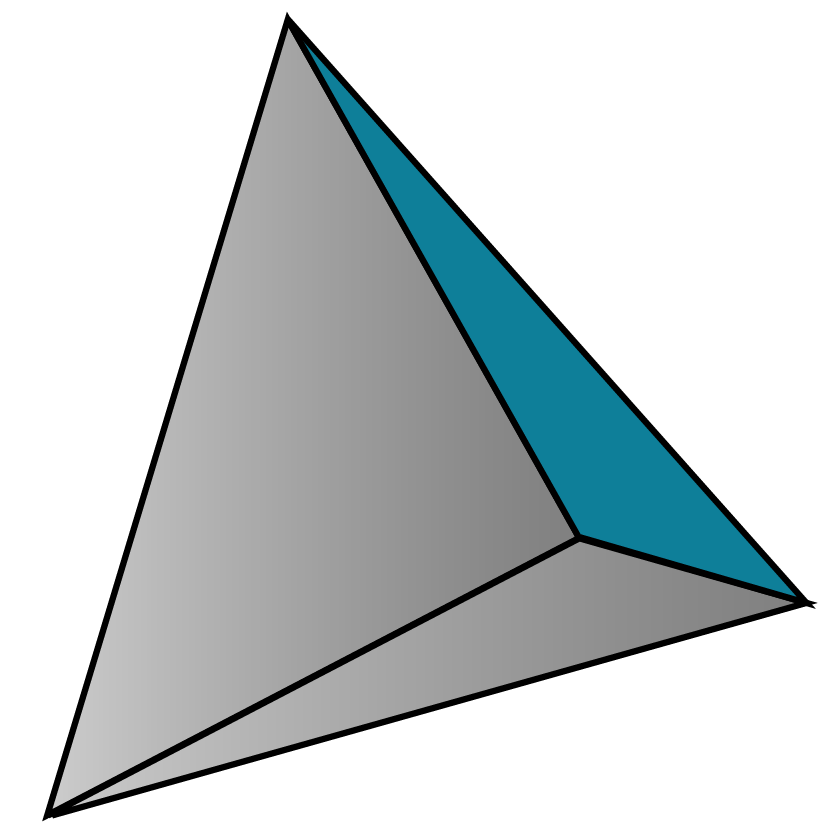## What is a Tetrahedron?

A tetrahedron is a three-dimensional shape having all faces as triangles.

### Net of a tetrahedron

Let us do a small activity.

Take a sheet of paper.

You can observe two distinct nets of a tetrahedron shown below.

Copy this on the sheet of paper.

Cut it along the border and fold it as directed in the figure shown below.

The folded paper forms a tetrahedron.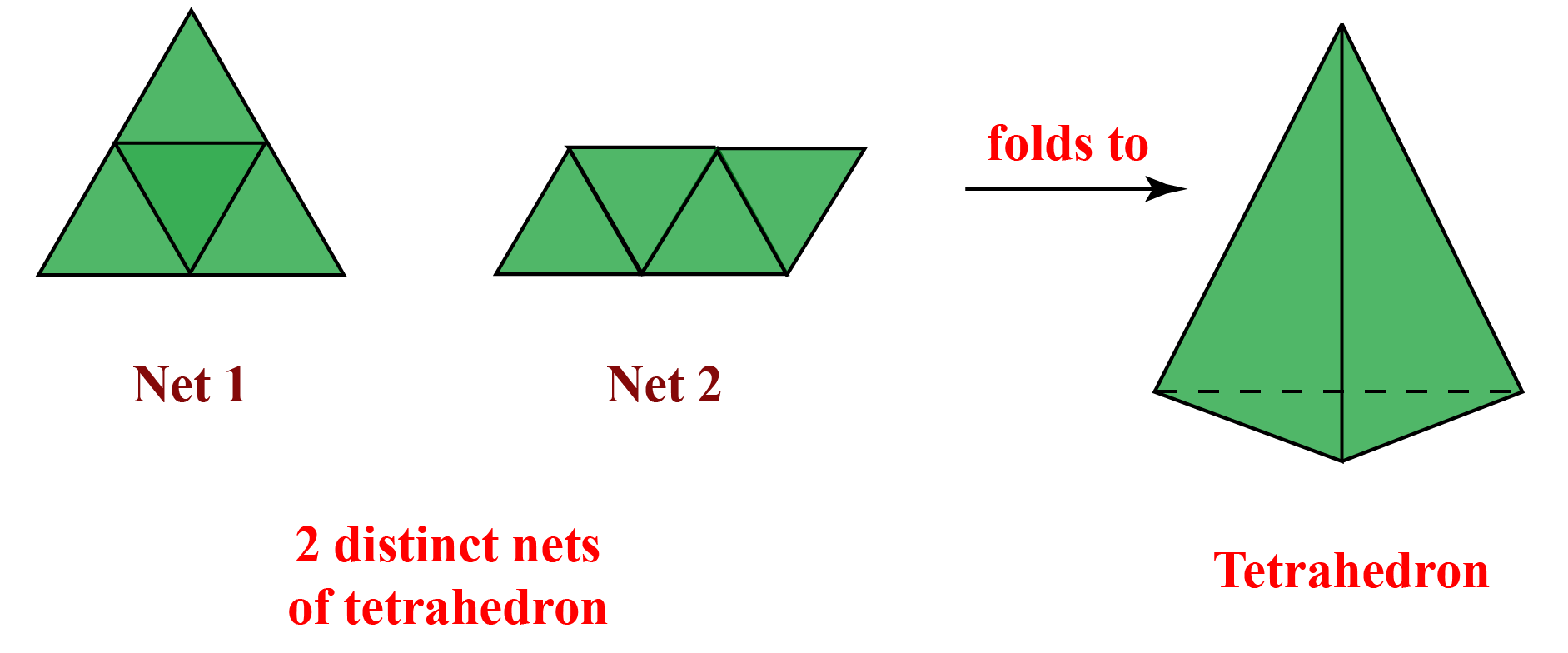The simulation below illustrates a tetrahedron in 3D.

Click on the edge of the tetrahedron and drag it around.

What do you see?

You will be able to view all the 4 faces of the tetrahedron as it rotates.

A regular tetrahedron has equilateral triangles as its faces.

Since it is made of equilateral triangles, all the internal tetrahedron angles will measure $$60^\circ$$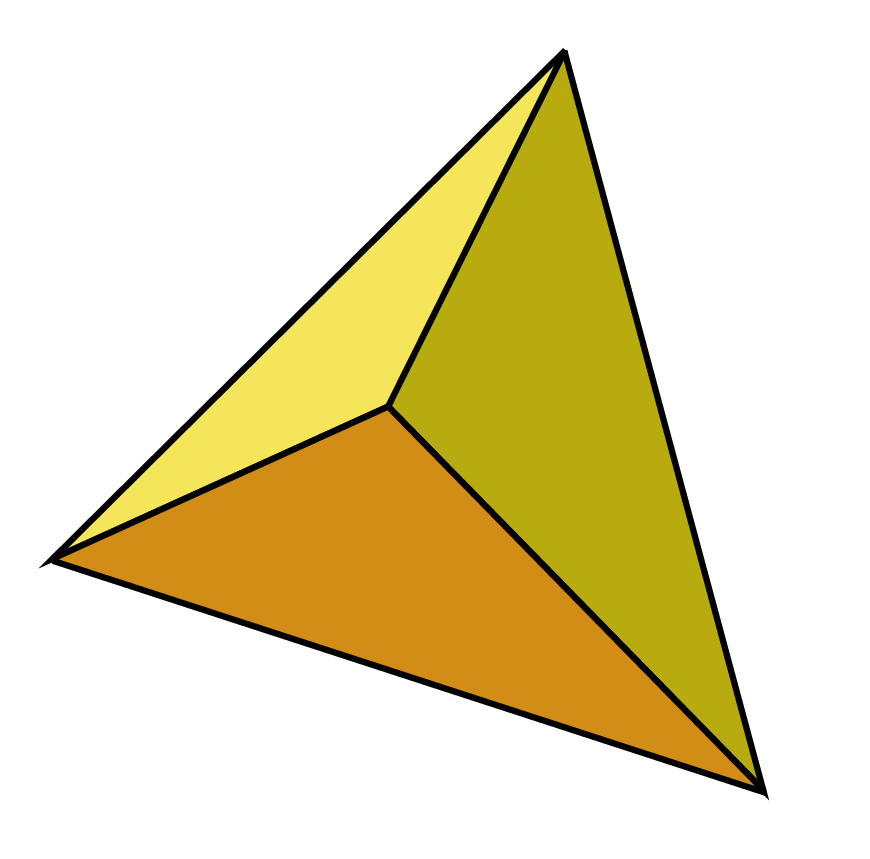An irregular tetrahedron also has triangular faces but they are not equilateral.

The internal tetrahedron angles in each plane add up to $$180^\circ$$as they are triangular.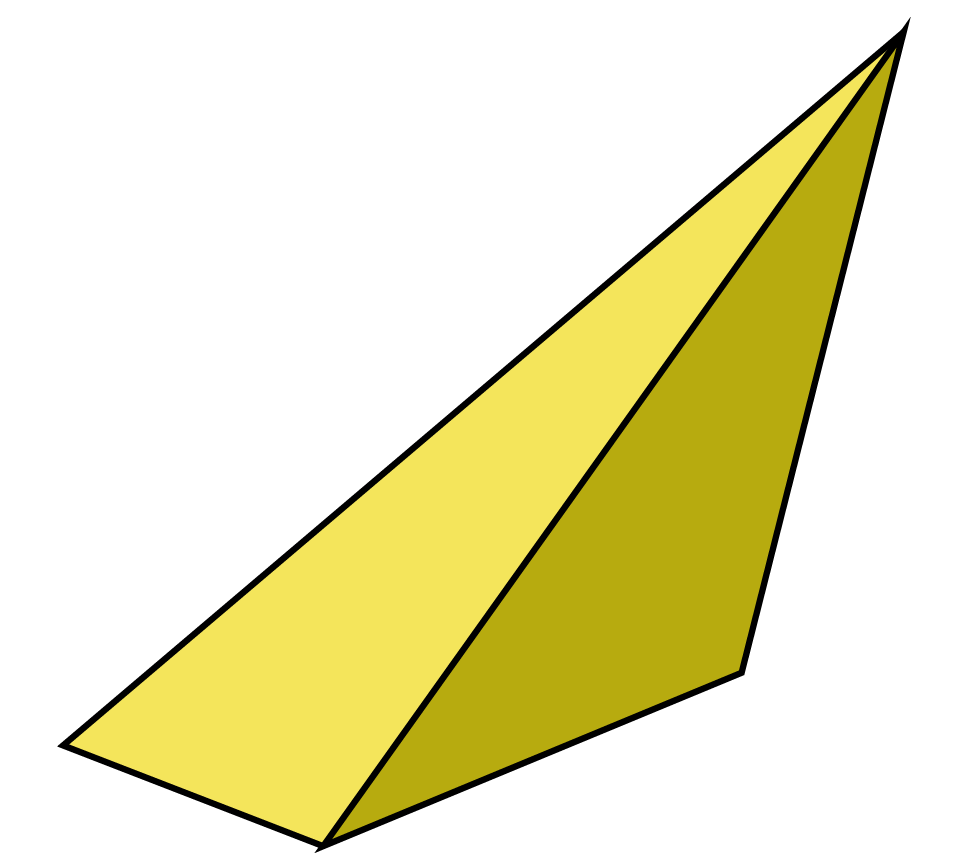Unless a tetrahedron is specifically mentioned as irregular, by default, all tetrahedrons are assumed to be regular tetrahedrons.

## Tetrahedron Properties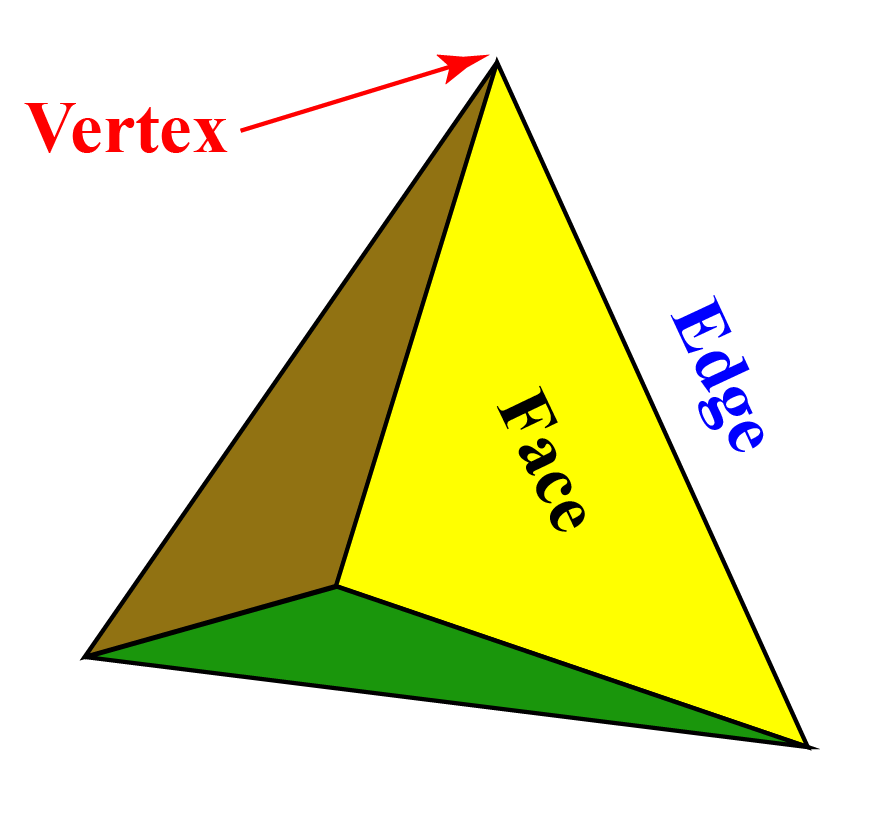• It has 4 faces, 6 edges and 4 corners.
• All four vertices are equally distant from each other.
• At each of its vertex, 3 edges meet.
• It has 6 planes of symmetry.
• Unlike other platonic solids, a tetrahedron has no parallel faces.
• A regular tetrahedron has equilateral triangles for all its faces.

## Tetrahedron Formula

Various tetrahedron formulas are listed below.

Consider a regular tetrahedron made of equilateral triangles of side $$s$$.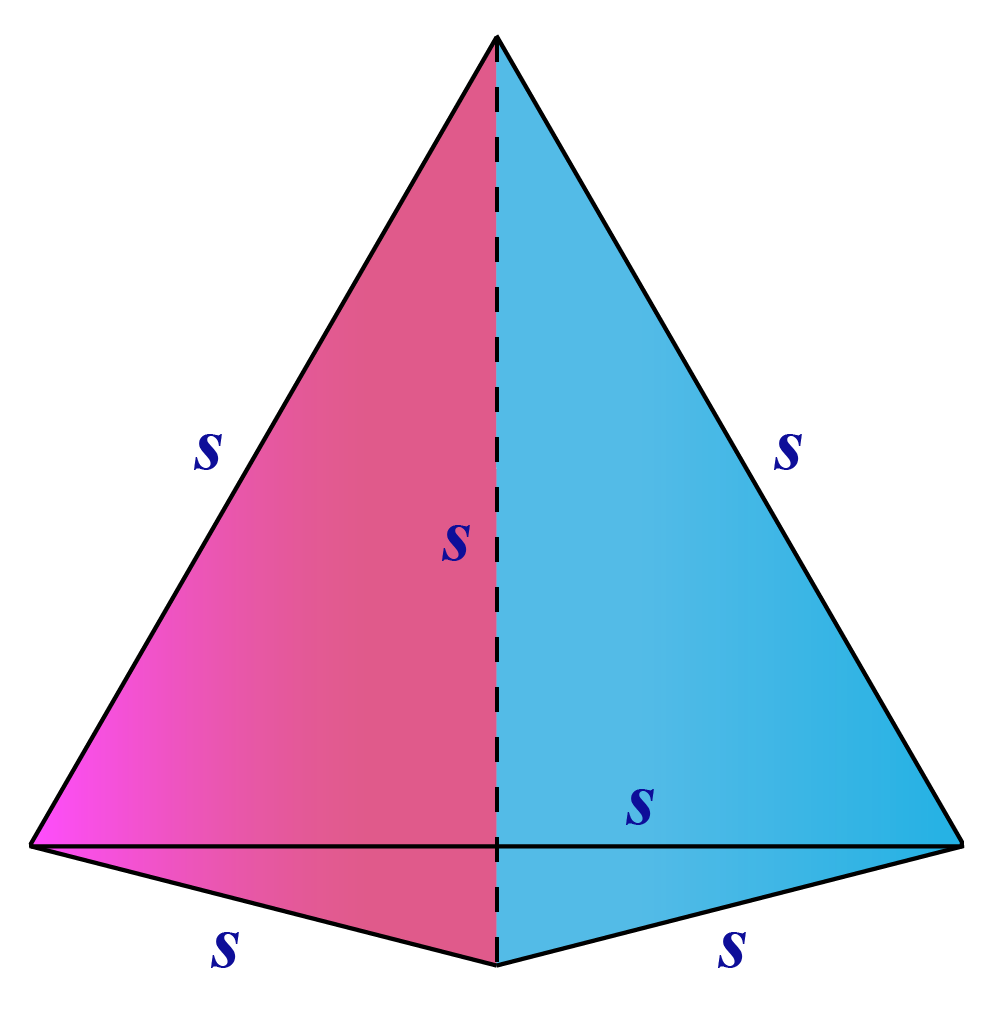Tetrahedron Volume:

 $$\text{Volume} = \frac{s^3}{6\sqrt{2}}$$

Total Surface Area of a Tetrahedron:

 $$\text{TSA} = \sqrt{3} \:s^2$$

Area of one face of a Tetrahedron:

 $$\text{ Area of a face } = \frac {\sqrt{3}}{4}s^2$$

Slant Height 's' of a Tetrahedron:

 $$\text{ Slant height} = \frac {\sqrt{3}}{2}s$$

Altitude 'h' of a Tetrahedron:

 $$\text{ Altitude} = \frac {s\sqrt{6}}{3}$$

Use the tetrahedron calculator to find the volume and total surface area.

Enter the edge length in the calculator below.

Help your child score higher with Cuemath’s proprietary FREE Diagnostic Test. Get access to detailed reports, customised learning plans and a FREE counselling session. Attempt the test now.

## Solved Examples

 Example 1

Two congruent tetrahedrons are stuck together along its base to form a triangular bipyramid.

How many faces, edges and vertices does this bipyramid have?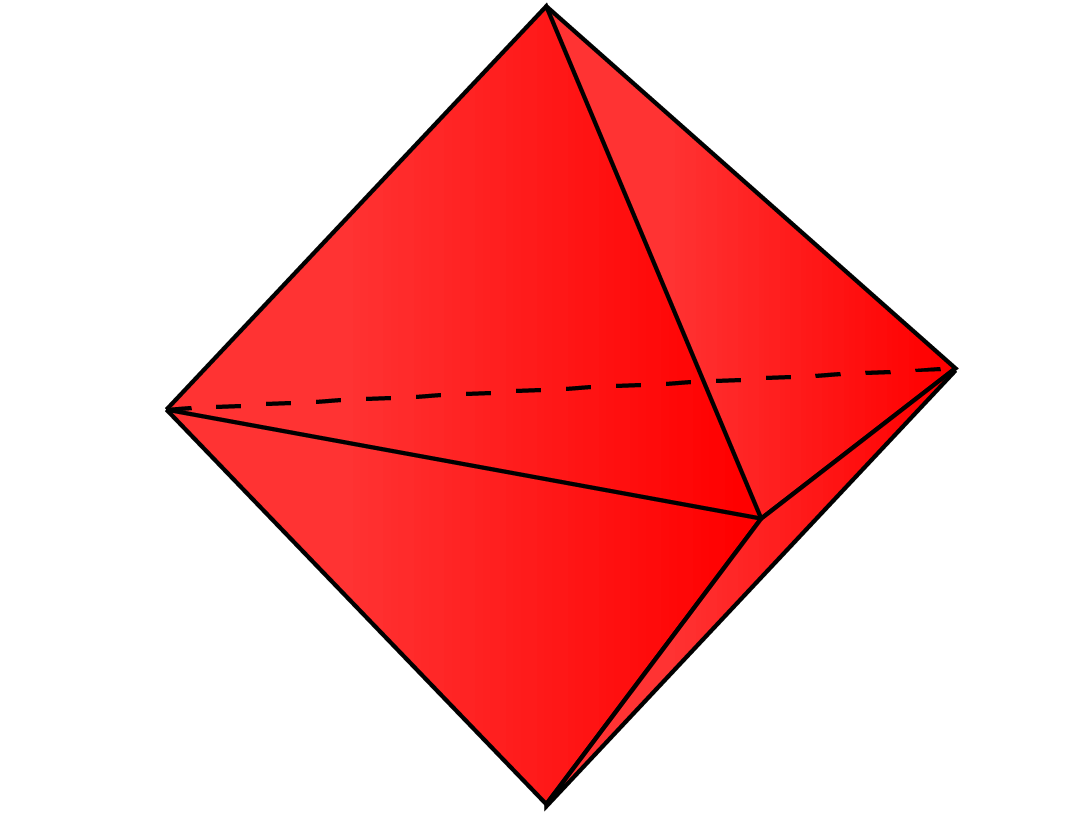Solution:

If we open up the above image to see the net of the triangular bipyramid, we can observe that: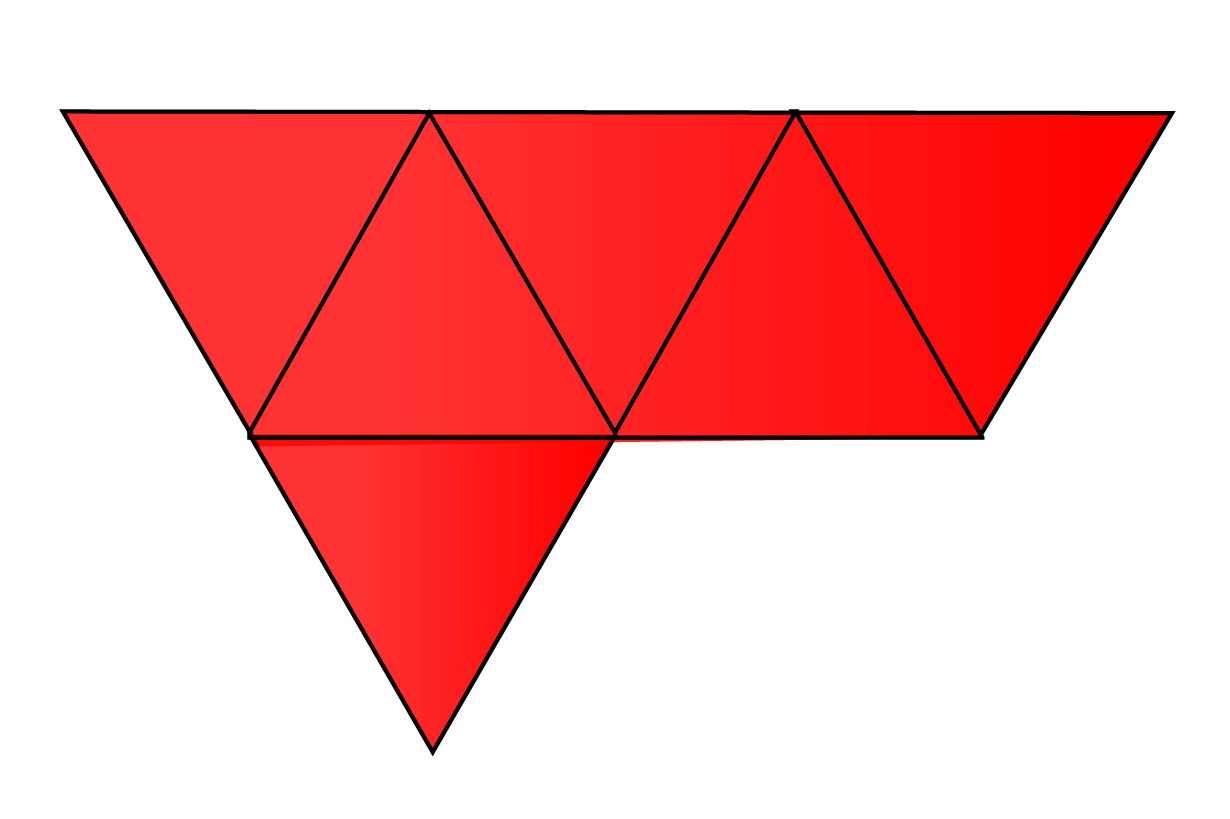There are 6 triangular faces, 9 edges and 5 vertices.

 Triangular bipyramid has 6 triangular faces, 9 edges and 5 vertices.
 Example 2

Find the volume of a regular tetrahedron with side length measuring 5 units.

(Round off the answer to 2 decimal places)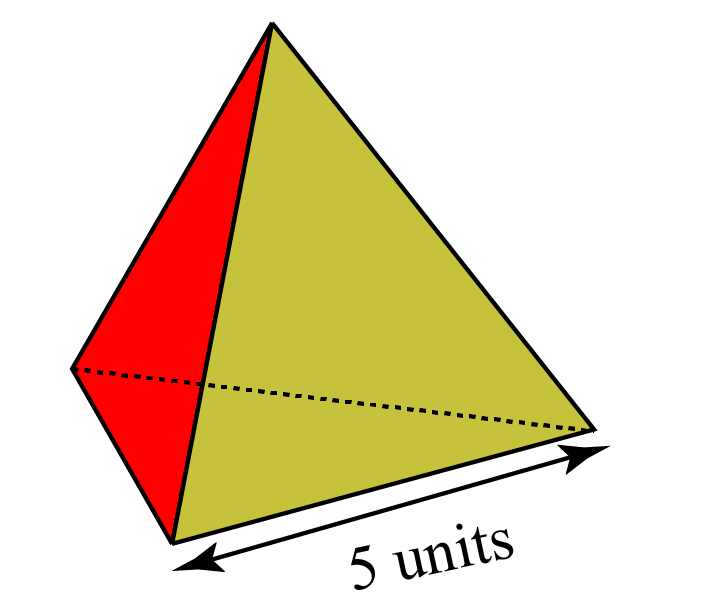Solution:

We know that tetrahedron volume whose side $$s$$ is:

\begin{align}\text{Volume} = \frac{s^3}{6\sqrt{2}}\end{align}

Substituting $$s$$ as 5 we get

\begin{align} \text{Volume} &= \frac{5^3}{6\sqrt{2}} \\\\ &=\frac{125}{8.485} \\\\ &\approx 14.73 \end{align}

 Volume of the tetrahedron is 14.73 units3
 Example 3

Each edge of a regular tetrahedron is of length 6 units.

Find its total surface area.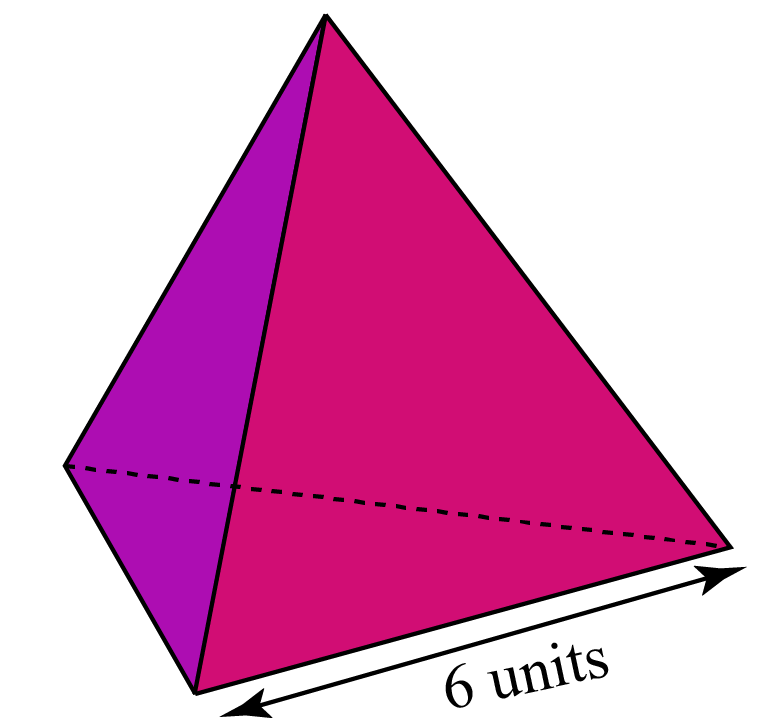Solution:

The total surface area of a regular tetrahedron of side $$s$$

$$\text{TSA} = \sqrt{3} \:s^2$$

Substituting s = 6,  we get

\begin{align} &\sqrt{3}\times\:6^2 \\\\ &= \sqrt{3} \times 6 \times 6\\ &= 62.35 \end{align}

 Total Surface Area = 62.35 units2
 Example 4

The sum of the length of the edges of a regular tetrahedron is 60 units.

Find the surface area of one of its faces.

Solution:

We know that a regular tetrahedron has 6 edges.

Therefore, the length of each edge is:

\begin{align}\frac{60}{6} = 10 \text{ units}\end{align}

Surface area of one face of the tetrahedron:

\begin{align}\text{ Area of a face } = \frac {\sqrt{3}}{4}s^2 \end{align}

Substituting s = 10, we get:

\begin{align} &\frac{\sqrt{3}}{4}10^2 \\\\ &= \frac{\sqrt{3}}{4} \times 10 \times 10\\ &=25\sqrt{3} \\ &=43.30 \end{align}

 Surface Area of one of its face = 8.66 units2
 Example 5

For what measure of the edge, a tetrahedron's total surface area is equal to its volume?

Solution:

We know that $$\text{TSA} = \sqrt{3} \:s^2$$ and \begin{align}\text{Volume} = \frac{s^3}{6\sqrt{2}}\end{align}

If TSA = Volume, we can say that:

$$\sqrt{3} \:s^2 = \frac{s^3}{6\sqrt{2}}$$

Solving for s, we have

\begin{align} \frac{s^3}{6\sqrt{2}}&= \sqrt{3} \:s^2 \\\\ \frac{s^3}{s^2} &= 6 \times \sqrt{3} \times \sqrt{2}\\ s&=6\sqrt{6} \end{align}

 Edge length of a tetrahedron is $$6\sqrt6$$

CLUEless in Math? Check out how CUEMATH Teachers will explain Tetrahedron to your kid using interactive simulations & worksheets so they never have to memorise anything in Math again!

Explore Cuemath Live, Interactive & Personalised Online Classes to make your kid a Math Expert. Book a FREE trial class today!

## Practice Questions

Here are a few activities for you to practice.Challenging Questions
1.  A new shape is formed by aligning the face of a tetrahedron exactly over one triangular face of the square pyramid. How many vertices, edges and faces will the new shape have?
2. Rody has a tent which is shaped like a regular tetrahedron. The volume of the tent is 100 m3 and the height is 6 m. What would be the edge length of his tent?

IMO (International Maths Olympiad) is a competitive exam in Mathematics conducted annually for school students. It encourages children to develop their math solving skills from a competition perspective.

## 1. What is tetrahedron?

A tetrahedron is a platonic solid having triangles as its faces.

## 2. What are the properties of a tetrahedron?

The properties of a tetrahedron are:

• It has 4 faces, 6 edges and 4 corners.
• All four vertices are equally distant from each other.
• Unlike other platonic solids, tetrahedron has no parallel faces.
• A regular tetrahedron has all its faces as equilateral triangles.
• At each vertex of a tetrahedron, 3 edges meet.
• A tetrahedron has 6 planes of symmetry.

## 3. How many tetrahedrons are in a cube?

There are 5 tetrahedrons in a cube.

The centre tetrahedron is regular and the others are irregular.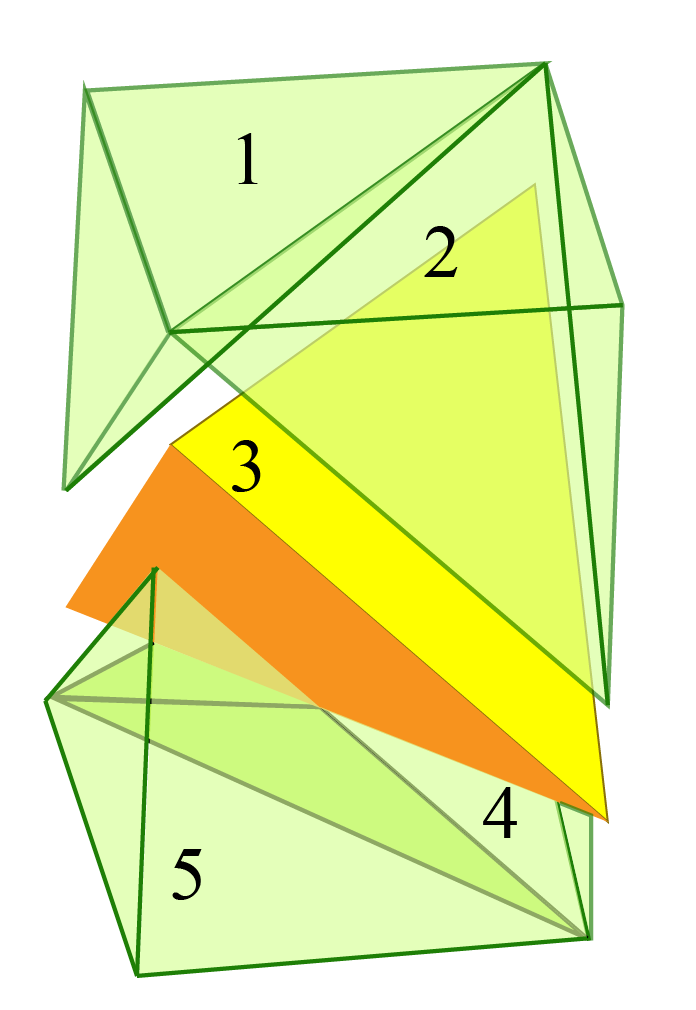More Important Topics
Numbers
Algebra
Geometry
Measurement
Money
Data
Trigonometry
Calculus